# Equation Worksheets

#### What are Equations?

To put it simply, an equation is a statement in mathematics that says that two things are equal. It has an equals sign "=" like this: 2 + 3 = 5. In algebra, the definition of an equation is 'a mathematical statement that consists of an equal symbol between two algebraic expressions that have the same value.' In math, the most common and basic algebraic equations, there are one or more variables. Let's look at an example: 4x + 10 = 20 is an equation in which 4x + 10 and 20 are two expressions separated by an 'equal' sign. In an algebraic expression, the right-hand side is equal to the left-hand side. Here, for example, 4x + 10 is the expression on the left-hand side, which is equal to the expression 20 on the right-hand side. The process of finding the value of the variable is called solving the equation.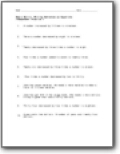###### Writing Number Sentences as Equations

Example problem: John has seven dollars. He needs x dollars more to buy a game that costs twenty five dollars.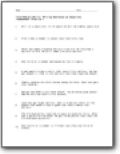###### Intermediate Skills

These are a step up from the previous series of worksheets. Example: A man wants to buy a shirt that costs fifty dollars. He has got amount x, that is ten dollars less than required amount.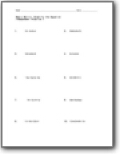###### Simplify the Equation

You will simplify linear equations to the lowest form. Example: 3k - 4 + 18 + 13k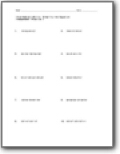###### Simplifying Slightly Complex Equations

You will drive down equations and even work with negative numbers. Example: 3p4 - 21p2 - 13p3 - 5p4 + 15p2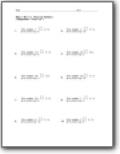###### Missing Numbers

You will fill in missing values to complete number sentences. Example: The number 34___ 0 is divisible by 10.